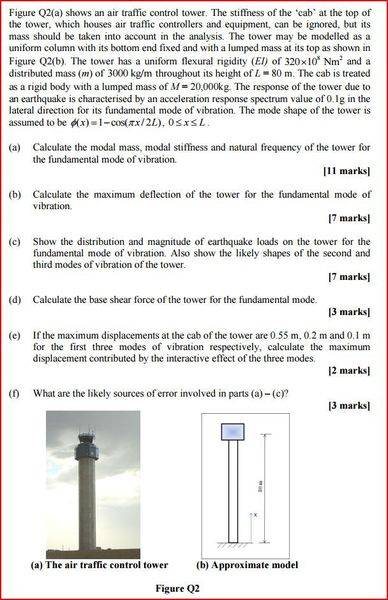# MDOF Earthquake Response

thethinwhiteduke

## Homework Statement

The question is featured in the image below. I know the methods to calculate modal mass, stiffness etc, I'm just not too sure how to model both the distributed and lumped masses together.## Homework Equations

Modal Mass, M* = ∫m.φ^2(x) dx

## The Attempt at a Solution

Say for instance, that the tower had no lumped mass at the top, so we only had to consider the distributed load (m = 3000 kg/m), the solution would be:
M* = m∫φ^2(x) dx (limits of integration are from 0 to L(=80m))
∫φ^2(x) dx = ∫(1-cos((πx)/(2L))^2 dx = L - 4L/π + L/2
M* = 3000 x (80 - 320/π +40) = 54423kg

My issue is not knowing how to combine the distributed and lumped masses together for this calculation. Any help is greatly appreciated